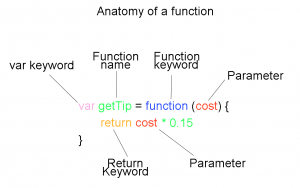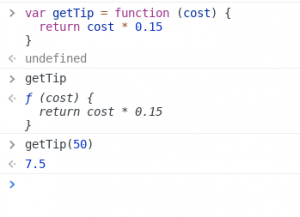# How to Write Your First Function In Javascript

#### Functions are the basic building block of programming

Now for the real meat of programming, functions. Functions are named sections of code that combine operations to perform a certain procedure. Functions can accept inputs and may return a value (an output). In the previous post, we started a bank and our first customer, John, deposited \$500. Whenever John made a transaction, we had to add or subtract the transaction from his balance manually. This is a perfect use case for a function. By the end of this post, you should understand how to write a function that can do exactly that.

#### A very basic functionLet’s start by making a very basic function that calculates a tip for us. Given we want to always tip an even 15%, this is an extremely simple function to write. To calculate this in the console would just be `cost * .15`, but now, let’s make a function. We can declare a function with the var keyword, just like we declared strings, arrays, and objects. The var keyword is followed by the function name, an equal sign and then the function’s body. Let’s call our tip calculator function, “getTip”. Since our tip is based on the cost of a service or meal, our function will need a way to accept that value as an input. Parameters are used to provide inputs to functions and we will need to input the meal cost, so let’s make a parameter called “cost”. Now, our function would look like this: `var getTip = function (cost) { return cost * 0.15 }`. The return keyword causes whatever follows it on the same line to be “outputted” from the function, but the return keyword also stops further execution of code. Try creating this function in the console (see how to open here). After the function is declared, it’s stored under the named variable you assigned it to. So, if you used getTip, you can type getTip, followed by enter to see that it has successfully been declared as a function. This will not execute the function, but simply display it in the console.

#### Invoke a functionNow to invoke a function, we enter the function’s name followed by a set of parentheses. Inside the parentheses, we place the value (or values) of our parameters. So, to calculate a tip for a \$50 meal, we would use `getTip(50)`. This will “return” (“return” is the output of a function) us the tip amount, or in this case, 50 * 0.15, which is 7.5.

#### Bank deposit function

Our getTip function takes a parameter “cost”, performs a calculation using this parameter and then returns a value; however, not all functions must return a value. Some functions can perform an action and modify an existing object, but return nothing. In a previous post we used a bank as an example, let’s use that example again. First declare the bank object, `var bank = {}`, and then we can add John’s account directly `bank.john = { accountType: "savings", balance: 500 }`. This is slightly different than how the account was created in the previous post, but it does the same thing. Now, if we type bank and hit enter, we should see our bank object with John’s account on it. Great, our bank is loaded. Next, let’s create a function named deposit, that will make a deposit to John’s bank account. We can name this function “deposit”, and it will take the balance in John’s account and add to it whatever amount is passed into the parameter “amount”. Thus, it would look like:

```var deposit = function (amount) {
bank.john.balance += amount
}```

We timed that perfectly, as John just won the lottery and is here to deposit his \$1,000,000 winnings. Let’s give it a try `deposit(1000000),` and now we need to check John’s account balance, `bank.john.balance`. Wow, that worked great, John’s balance is now \$1,000,500, and we didn’t have to do any manual update to his account balance. However, there is a problem here: this function works great if we only have one customer, but most banks have multiple customers, and they will not be happy if every time they each make a deposit, it winds up in John’s bank account. Fortunately, there are a lot of great solutions for this problem, and in future posts, we will cover fun and useful concepts like classes and inheritance that solve this and similar problems.

#### Practice

OK, in the comment sections, see if you can create functions to do some of the following:

• Multiply a number by 2
• Square a number
• Make a greeting function that takes someones name and returns “Hello, [name here].”
• Function to withdraw from an account
• A function to add interest into a bank account
• Or another problem you can think of